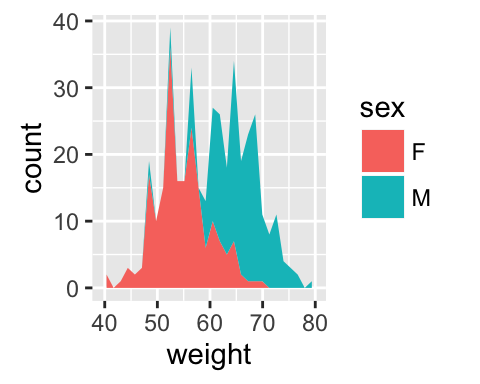# ggplot2 area plot : Quick start guide - R software and data visualization

This R tutorial describes how to create an area plot using R software and ggplot2 package. We’ll see also, how to color under density curve using geom_area.

The function geom_area() is used. You can also add a line for the mean using the function geom_vline.ggplot2 geom_area - R software and data visualization

# Prepare the data

This data will be used for the examples below :

``````set.seed(1234)
df <- data.frame(
sex=factor(rep(c("F", "M"), each=200)),
weight=round(c(rnorm(200, mean=55, sd=5),
rnorm(200, mean=65, sd=5)))
)
``````##   sex weight
## 1   F     49
## 2   F     56
## 3   F     60
## 4   F     43
## 5   F     57
## 6   F     58``````

# Basic area plots

``````library(ggplot2)
p <- ggplot(df, aes(x=weight))
# Basic area plot
p + geom_area(stat = "bin")
# y axis as density value
p + geom_area(aes(y = ..density..), stat = "bin")
p + geom_area(stat = "bin", fill = "lightblue")+
geom_vline(aes(xintercept=mean(weight)),
color="blue", linetype="dashed", size=1)``````ggplot2 geom_area - R software and data visualization

# Change line types and colors

``````# Change line color and fill color
p + geom_area(stat ="bin", color="darkblue",
fill="lightblue")
# Change line type
p + geom_area(stat = "bin", color= "black",
fill="lightgrey", linetype="dashed")``````ggplot2 geom_area - R software and data visualization

Read more on ggplot2 line types : ggplot2 line types

# Change colors by groups

## Calculate the mean of each group :

``````library(plyr)
mu <- ddply(df, "sex", summarise, grp.mean=mean(weight))
``````##   sex grp.mean
## 1   F    54.70
## 2   M    65.36``````

## Change fill colors

Area plot fill colors can be automatically controlled by the levels of sex :

``````# Change area plot fill colors by groups
ggplot(df, aes(x=weight, fill=sex)) +
geom_area(stat ="bin")
# Use semi-transparent fill
p<-ggplot(df, aes(x=weight, fill=sex)) +
geom_area(stat ="bin", alpha=0.6) +
theme_classic()
p
p+geom_vline(data=mu, aes(xintercept=grp.mean, color=sex),
linetype="dashed")``````ggplot2 geom_area - R software and data visualization

It is also possible to change manually the area plot fill colors using the functions :

• scale_fill_manual() : to use custom colors
• scale_fill_brewer() : to use color palettes from RColorBrewer package
• scale_fill_grey() : to use grey color palettes
``````# Use custom color palettes
p+scale_fill_manual(values=c("#999999", "#E69F00"))
# use brewer color palettes
p+scale_fill_brewer(palette="Dark2")
# Use grey scale
p + scale_fill_grey()``````ggplot2 geom_area - R software and data visualization

Read more on ggplot2 colors here : ggplot2 colors

# Change the legend position

``````p + theme(legend.position="top")
p + theme(legend.position="bottom")
p + theme(legend.position="none") # Remove legend``````ggplot2 geom_area - R software and data visualization

The allowed values for the arguments legend.position are : “left”,“top”, “right”, “bottom”.

Read more on ggplot legends : ggplot2 legends

# Use facets

Split the plot in multiple panels :

``````p<-ggplot(df, aes(x=weight))+
geom_area(stat ="bin")+facet_grid(sex ~ .)
p
p+geom_vline(data=mu, aes(xintercept=grp.mean, color="red"),
linetype="dashed")``````ggplot2 geom_area - R software and data visualization

Read more on facets : ggplot2 facets

# Contrasting bar plot and area plot

An area plot is the continuous analog of a stacked bar chart. In the following example, we’ll use diamonds data set [in ggplot2 package]:

``````# Load the data
data("diamonds")
p <- ggplot(diamonds, aes(x = price, fill = cut))
``````##   carat       cut color clarity depth table price    x    y    z
## 1  0.23     Ideal     E     SI2  61.5    55   326 3.95 3.98 2.43
## 2  0.21   Premium     E     SI1  59.8    61   326 3.89 3.84 2.31
## 3  0.23      Good     E     VS1  56.9    65   327 4.05 4.07 2.31
## 4  0.29   Premium     I     VS2  62.4    58   334 4.20 4.23 2.63
## 5  0.31      Good     J     SI2  63.3    58   335 4.34 4.35 2.75
## 6  0.24 Very Good     J    VVS2  62.8    57   336 3.94 3.96 2.48``````
``````# Bar plot
p + geom_bar(stat = "bin")``````ggplot2 geom_area - R software and data visualization

``````# Area plot
p + geom_area(stat = "bin") +
scale_fill_brewer(palette="Dark2") ``````ggplot2 geom_area - R software and data visualization

# Coloring under density curve using geom_area

``````dat <- with(density(df\$weight), data.frame(x, y))
ggplot(data = dat, mapping = aes(x = x, y = y)) +
geom_line()+
geom_area(mapping = aes(x = ifelse(x>65 & x< 70 , x, 0)), fill = "red") +
xlim(30, 80)``````ggplot2 geom_area - R software and data visualization

# Infos

This analysis has been performed using R software (ver. 3.2.4) and ggplot2 (ver. 2.1.0)